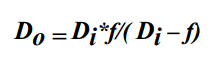Subject:
Mathematics

Topics: AlgebraRational Functions
Common Core State Standard: F-IF.7d,
Knowledge and Skills:

· Can graph a rational function

Lesson:

Procedure: This activity is best done with students working individually or in teams of two.

Distribute the handout and ensure students understand what they are being asked to do.

To create the graphs, students will need to solve the given equation for Do:Once the graphs are created you may wish to discuss some of its interesting points, such as what happens when Di is less than f, equal to f, or equal to 2f.

### Lens Sculptors

Memorandum

From: Senior Optical Scientist
Subject: Lens characteristics

You all know that the distance from an object to a lens (Do) is related to the distance from the lens to the image (Di ) by this equation:

1/Do + 1/Di = 1/f

where “f” is the focal length of the lens.

For the three new lenses we are about to release, the marketing department wants to have graphs that show how the image distance (Di ) depends on the object distance (Do).

The focal lengths of these three new lenses are 25 mm, 35 mm, and 50 mm.

One other point: for the 50 mm. lens, we need to know what the object distance would be for image distances of 58, 60, and 62 mm.

Have this on my desk by tomorrow morning, please.

A. Leeuwenhoek